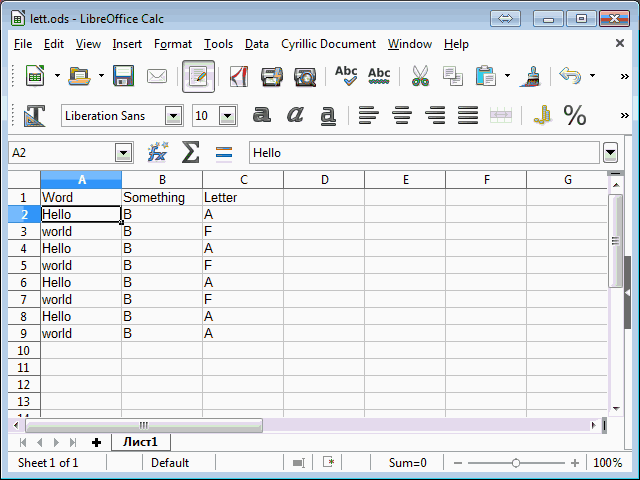# Count rows and search for letter

Is there a ways in different worksheets and in a particular column is searched for a letter and the found are given in percent?

Example

``````     A         B      C
1   Hello      B      A
2   world      B      F
``````

So is the table built and i want count in all spreadsheets in column C for A , for F or for A. The found letter should imaging in percent for all filled line in spreadsheet.
Hope is to understand, to explain is ever so hard.

(Table edited by @Lupp)

Understand too hard. “percent” of what? From the total number of characters in the workbook? Do you need calculate the spaces? What do you have in mind when wrote “in column C for A , for F or for A”?

I think you need a PivotTableTo do so actually accross an arbitrary number of sheets is not possible by a single formula, because the very few functions accepting ‘cuboids’ as parameters are not appropriate to fulfil the expectations. You would need something like

``````=COUNTIF(\$SheetFirst.\$C\$1:\$SheetLast.\$C\$40;"A")/COUNTA(\$SheetFirst.\$C\$1:\$SheetLast.\$C\$40)
``````

COUNTA is capable of processing cuboids. COUNTIF is not.

Possible workarounds:

1. Rework the formula to explicitly reference each sheet on its own parameter position. (Fix limit for number of sheets!)
2. Use (at least) one helper column in each sheet, allowing to accomplish the task using SUM and COUNTA (or similar).
3. Code a user function for the purpose.

Hello,

yes found same. Thanks. Now it work.

``````=COUNTIF(\$'2003'.M2:\$'2003'.M50;"B")/COUNTA(\$'2003'.A1:A50)
``````

Silvio

This is not going accross many sheets.
The reference used with COINTIF is unnecessarily complicated.